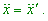V.5 No 2 1 Supplement 1Supplement 1 Some additional aspects of the analysis of black hole conception S. B. Karavashkin and O.N. Karavashkina Special Laboratory for Fundamental Elaboration SELF 187 apt., 38 bldg., Prospect Gagarina, Kharkov, 61140, Ukraine phone +38 (057) 7370624; e-mail: selftrans@yandex.ru , selflab@mail.ru http://selftrans.narod.ru/SELFlab/index.html http://selftrans.narod.ru/index.html   After our paper was advertised, it was actively discussed on several forums. At the same time, as if in response to our notice about sensations for one day, NASA has disseminated a release that they have discovered 21 black hole at once, and globally recognised institutions confirmed this discovery true. In this connection, we thought necessary to add some details to our previous study and to analyse the NASA’s discovery.   Let us begin with the doubt of one colleague that Einstein grounded his GR not on the principle of equivalence of inertial and gravity mass but on the principle of equivalence of physical processes in inertial and non-inertial reference frames. True, in the page 14 of our work we analysed this issue quite enough and gave citations from Einstein and his followers. They all unambiguously state, under the principle of equivalence of inertial and gravity mass Einstein meant namely the equivalence of inertial and non-inertial reference frames. This principle is reflected also in relativistic modelling of processes. In particular, when Landau derives Schwarzschild’s metric, he points that he fully free substitutes the reference frame, irrespectively of, whether the frame was inertial or not. This we also mentioned in our study, in page 7. The same, Einstein in his work “The problem of particles in general theory of relativity”, doing not thinking of the physical meaning of introduced values, writes down in Schwarzschild’s solution a new value of radius [1, p. 428] as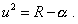(1)
 (we write this formula in the symbols used by Schwarzschild). With it, Schwarzschild’s solution takes the following form: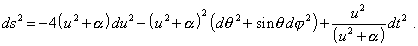(2)
 “The new values g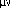that we yielded in this way appear to be the regular functions with all values of variables … The solution free of singularities we can now think as the mathematical description of a particle (neutron either neutrino). A salient feature of the theory which we develop is, it describes the space with the help of two sheets. The spatially finite ‘bridge’ connecting these sheets describes an electrically neutral particle” [1, p. 428]. With it, the parameter u was and remained an abstract non-measured parameter irrelevant to the real space. The measured parameter is even not R but, according to Schwarzschild’s derivation [2, p. 205],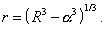(3)
 In such approach to the mathematical modelling, any phantasmagoric solutions are possible, of course, but symbols introduced artificially remain such and predestine the next difficulties. From this view, there arises a simple question. Does there really exist Schwarzschild radius in the form in which it is described by relativists - as some radius R =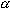at which the metric becomes singular, due to which there arises some event horizon which neither substance nor light can surmount? “Everything that crosses the horizon will be squashed, pressed ‘into nothing’ ” [3, p. 103]. However strange it can seem with the abundant literature which more than 90 years was published on different versions of Schwarzschild’s geometry, with all generously funded projects in whose frames the astronomers attempt to reveal the celestial bodies whose substance to be compressed to this radius, - the answer to this question still is negative. Actually, the final Schwarzschild’s solution in spherical coordinates is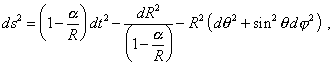(4)
 where R  is determined by (3). As we already said, this metric has a singularity at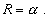(5)
 But R is not a measured parameter. As we also said above and as follows from the statement of problem in Schwarzschild’s derivation, the measured parameter is r. So (5) in the measured parameters will take the following form: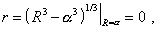(6)
 and it follows from (3) that as the measured parameter r cannot be negative, the condition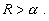(7)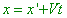(a)